IBPS PO Quantitative Aptitude Quiz

With the increasing level in exams, quantitative aptitude has become the ineluctable hitch. Generally, questions asked in this section are calculative and lengthy that consumes your time. This subject can do wonders if you always keep a check on your accuracy, speed and time. Accuracy is what matters the most. To help you prepare the section we have provided a well-defined IBPS PO Prelims Study Plan. You can also Prepare from the study notes to clear your basic concepts. Attempt this quantitative aptitude quiz and check your performance for the upcoming IBPS PO Prelims Study Plan 2019. Following is the quiz of 13th August, that inculcate the important topic from Quadratic Equations.

Directions (1-15): In each of these questions, two equations (I) and (II) are given. You have to solve both the equations and give answer

Q1.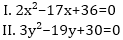if x > y
if x < y
if x = y or no relation can be established between x and y
if x ≤ y
if x ≥ y

Q2.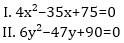if x > y
if x < y
if x = y or no relation can be established between x and y
if x ≤ y
if x ≥ y

Q3.if x > y
if x < y
if x = y or no relation can be established between x and y
if x ≤ y
if x ≥ y

Q4.if x > y
if x < y
if x = y or no relation can be established between x and y
if x ≤ y
if x ≥ y

Q5.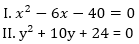if x > y
if x < y
if x = y or no relation can be established between x and y
if x ≤ y
if x ≥ y

Q6.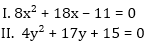if x > y
if x < y
if x = y or no relation can be established between x and y
if x ≤ y
if x ≥ y

Q7.if x > y
if x < y
if x = y or no relation can be established between x and y
if x ≤ y
if x ≥ y

Q8.if x > y
if x < y
if x = y or no relation can be established between x and y
if x ≤ y
if x ≥ y

Q9.if x > y
if x < y
if x = y or no relation can be established between x and y
if x ≤ y
if x ≥ y

Q10.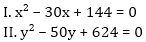if x > y
if x < y
if x = y or no relation can be established between x and y
if x ≤ y
if x ≥ y

Q11.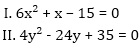if x > y
if x < y
if x = y or no relation can be established between x and y
if x ≤ y
if x ≥ y

Q12.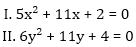if x > y
if x < y
if x = y or no relation can be established between x and y
if x ≤ y
if x ≥ y

Q13.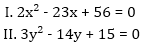if x > y
if x < y
if x = y or no relation can be established between x and y
if x ≤ y
if x ≥ y

Q14.if x > y
if x < y
if x = y or no relation can be established between x and y
if x ≤ y
if x ≥ y

Q15.if x > y
if x < y
if x = y or no relation can be established between x and y
if x ≤ y
if x ≥ y